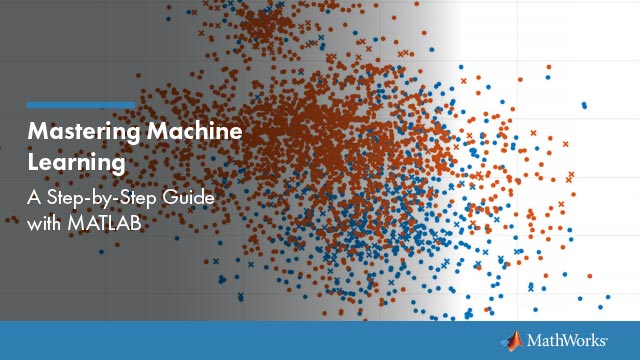Ready to start applying machine learning with MATLAB®? Get started with a MATLAB machine learning example presented in an easy-to-follow tutorial format.

Get this ebook, download the code, and step through a hands-on machine learning tutorial that helps you master machine learning techniques. The MATLAB machine learning example, a heart sounds classifier, takes you from loading data to deploying a trained model.

• Access and explore data
• Preprocess data using stepwise regression and regularization for feature selection
• Develop predictive models with classification algorithms, including decision trees, K-nearest neighbors, and naive Bayes
• Optimize model performance and tune hyperparameters by using grid search and Bayesian optimization
• Deploy analytics to desktops, enterprise IT systems, the cloud, and embedded systems